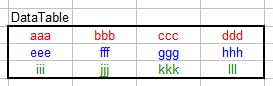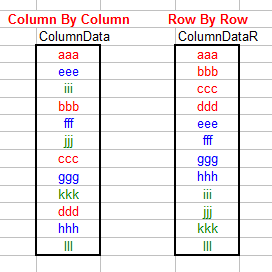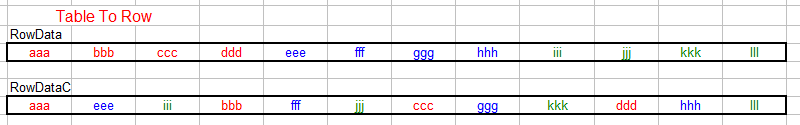Converting A Table To A Column

This page describes formulas and VBA code you can use to convert a two-dimensional table to a single column.Table To Column Or Row

Elsewhere on this web site, we examined formulas and code to convert a single column of data into a two-dimensional table of rows and columns. This page introduces the reverse of that: we'll look at formulas to convert a two-dimensional table to a single column or a single row. Moreover, we will look at formulas that will return the data from the table row-by-row (horizontally) or column-by-column (vertically). The data table we will use as an example for these formulas is shown below:Table To Column

First, we'll look at the formulas to convert the table to a single column. The data table has been assigned the defined name DataTable and the resulting column of data has been assigned the name ColumnData. (It is sufficient to name only the first cell, not the entire range.) Enter the following formula in the first cell of ColumnData and fill down for as many rows as there are elements (rows x columns) in DataTable:

=OFFSET(DataTable,MOD(ROW()-ROW(ColumnData),ROWS(DataTable)),TRUNC((ROW()-ROW(ColumnData))/ROWS(DataTable),0),1,1)

This will retrieve the values from DataTable in column-by-column order, working down then across. You can retrieve the values from DataTable in row-by-row order order, working across then down, with the following formula:

=OFFSET(DataTable,TRUNC((ROW()-ROW(ColumnDataR))/COLUMNS(DataTable),0),MOD(ROW()-ROW(ColumnDataR),COLUMNS(DataTable)),1,1)

This formula uses the defined name ColumnDataR to name the result column (or at least the first cell of the result column).

The results of both formulas are shown below. The column on the left returns the data from DataTable in column-by-column order. The column on the right returns the data from DataTable in row-by-row order:Table To Row

We can use similar formulas to transform DataTable into a single row. The following formula uses the defined name RowData to identify the resulting row of data. The first formula will retrieve the data from DataTable in row-by-row order, moving across then down. Enter the following formula in the first cell of RowData and fill across as many columns as there are elements (rows x columns) of DataTable.

=OFFSET(DataTable,TRUNC((COLUMN()-COLUMN(RowData))/COLUMNS(DataTable),0),MOD(COLUMN()-COLUMN(RowData),COLUMNS(DataTable)),1,1)

A similar formula can be used to transform DataTable into a row in column-by-column order, moving down the across. The formula below uses the defined name RowDataC to identify the result row (or at least the first cell of the result row) into which the values of DataTable are returned. Enter the following formula in the first cell of RowDataC:

=OFFSET(DataTable,MOD(COLUMN()-COLUMN(RowDataC),ROWS(DataTable)),TRUNC((COLUMN()-COLUMN(RowDataC))/ROWS(DataTable),0),1,1)

Fill this formula across the row for as many columns as there are values (rows x columns) in DataTable. The resulting rows of these formulas are shown below:This page last updated: 20-Nov-2008.

-->